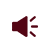• ISSN 1001-1455  CN 51-1148/O3
• EI、Scopus、CA、JST收录
• 力学类中文核心期刊
• 中国科技核心期刊、CSCD统计源期刊引用本文: 苏琼, 程月华, 吴昊. 爆炸荷载作用下UHPC板的弯曲损伤评估[J]. 爆炸与冲击.SU Qiong, CHENG Yuehua, WU Hao. Flexural damage assessment for UHPC panels under blast loadings[J]. Explosion And Shock Waves. doi: 10.11883/bzycj-2023-0160
 Citation: SU Qiong, CHENG Yuehua, WU Hao. Flexural damage assessment for UHPC panels under blast loadings[J]. Explosion And Shock Waves.## 爆炸荷载作用下UHPC板的弯曲损伤评估

##### doi: 10.11883/bzycj-2023-0160

###### 通讯作者: 程月华（1994－　），女，博士，yhcheng@tongji.edu.cn
• 中图分类号: O389;TU375.2

• 摘要: 为构建爆炸荷载作用下超高性能混凝土（UHPC）板弯曲损伤等级评估的压力-冲量（p-I）曲线：采用条带法进行截面分析，建立了考虑材料拉/压软化和塑性铰影响的UHPC简支单向板的非线性抗力方程和等效单自由度（ESDOF）理论模型；通过与六炮次爆炸实验中UHPC板的挠度时程，以及UFC 3-340-02和FHWA规范推荐方法的计算结果对比，验证了本文理论模型的可靠性；基于验证的ESDOF模型，构建了评估UHPC板的不同弯曲损伤等级的p-I曲线并开展了参数影响分析，提出并验证了UHPC板弯曲损伤评估的p-I曲线经验公式。结果表明：提高混凝土强度等级和钢筋屈服强度，增加受拉钢筋配筋率和板厚，以及减小净跨均可提升UHPC板的抗爆性能。
• 图  1  ESDOF模型及爆炸荷载简化

图  2  双线性理想弹-塑性抗力方程

Figure  2.  Bilinear ideal elastoplastic resistance function

图  3  实际简支构件及其对应的理想半跨对称梁模型

Figure  3.  Actual simply supported member and the corresponding half-span symmetric beam model

图  4  条带法截面分析示意图

Figure  4.  Schematic diagram of cross-sectional analysis by strip method

图  5  UHPC和钢筋本构模型[21-23]

Figure  5.  Constitutive models of UHPC and reinforcement[21-23]

图  6  非线性抗力方程的建立及荷载-质量转换系数与挠度关系

Figure  6.  Nonlinear resistance function and the relationship between KLM and deflection

图  7  UHPC单轴拉伸应力-应变曲线

Figure  7.  Uniaxial tensile stress-strain curve of UHPC

图  8  UHPC板的预测挠度时程与实验/数值仿真结果对比

Figure  8.  Comparisons of predicted deflection-time histories and experimental/simulated results of UHPC panels

图  9  UHPC-D4的预测挠度时程与实验结果对比

Figure  9.  Comparisons of predicted and experimental deflection-time histories of UHPC-D4

图  10  UHPC板的ESDOF模型预测挠度-时程与实验结果对比

Figure  10.  Comparisons of ESDOF model predicted deflection-time histories and experimental results of UHPC panels

图  11  基准板p-I曲线

Figure  11.  p-I diagrams for control panel

图  12  不同混凝土强度等级UHPC板的p-I曲线

Figure  12.  p-I diagrams for UHPC panel with different concrete strength grades

图  13  不同钢筋屈服强度UHPC板的p-I曲线

Figure  13.  p-I diagrams for UHPC panel with different yield strengths of reinforcement

图  14  不同纵筋配筋率UHPC板的p-I曲线

Figure  14.  p-I diagrams for UHPC panel with different longitudinal reinforcement ratios

图  15  不同厚度UHPC板的p-I曲线

Figure  15.  p-I diagrams for UHPC panel with different thicknesses

图  16  不同净跨UHPC板的p-I曲线

Figure  16.  p-I diagrams for UHPC panel with different clear spans

图  17  基于ESDOF模型分析生成的p-I曲线与经验公式预测结果对比

Figure  17.  Comapriosns between p-I diagrams from ESDOF model ananlysis and empirical formulae

表  1  简化爆炸荷载特征参数

 实验 试件 爆炸类型 pe/MPa te/ms Su等 UHPC-1 近场爆炸 7.122 0.28 UHPC-2 近场爆炸 12.59 0.20 UHPC-3 近场爆炸 18.52 0.16 Li等 UHPC-D4 近场爆炸 20.89 0.20 Mao等 A 远场爆炸 1.16 3 B 远场爆炸 2.488 1.9

表  2  UHPC板的尺寸、配筋及材料特性参数

Table  2.   Dimensions, reinforcement and material properties parameters of UHPC panels

 实验 L/mm b/mm h/mm ρt/% ρc/% fc/MPa Ec/GPa vf/% Su等 2000 1000 100 0.864 0.864 148 48.6 2 Li等 1800 1000 100 0.679 0.339 128.9 51.5 2 Mao等 3400 1300 100 3.4 0 170 54.8 2 实验 ft/MPa ft1/MPa ε1 εfrac fy Es Et εsu Su等 8.33 4.23 0.001 0.011 480 184 1.42 0.15 Li等 7.66 9.95 0.005 0.02 300 200 2 0.15 Mao等 10 10 0.004 0.01 500 200 2 0.15

表  3  ESDOF模型预测峰值挠度与实验/数值仿真结果对比

Table  3.   Comparisons of ESDOF model predicted and experimental/simulated maximum deflections

 实验 试件编号 实验值/mm 模拟值/mm UFC 3-340-02 FHWA 本文 预测值/mm 误差/% 预测值/mm 误差/% 预测值/mm 误差/% Su等 UHPC-1 27.86 27.44 27.73 −0.5 17.39 −37.6 26.42 −5.2 UHPC-2 − 38.90 41.89 7.6 24.92 −35.9 37.25 −4.2 UHPC-3 − 48.13 55.98 16.3 32.56 −32.3 47.67 −1.0 Li等 UHPC-D4 72 − 123.99 72.2 49.95 −30.6 73.11 1.5 Mao等 A 110 − 113.24 2.9 88.24 −19.8 111.44 1.3 B 210 − 193.32 −7.9 145.24 −30.8 213.46 1.6

表  4  UHPC板弯曲损伤p-I曲线经验公式相关参数

Table  4.   Parameters of the empirical formulae of flexural damage p-I diagrams for UHPC panels

 影响因素 参数 表达式 θ=2° θ=5° 基准板 pb0 106 127 Ib0 1214 2228 βb 1.634 1.642 fc/MPa，100≤fc≤250 ${\eta _{{f_{\text{c}}}}}$ 0.717 + 0.325 × (fc/150) − 0.042 × (fc/150)2 0.251 + 1.065 × (fc/150) − 0.319 × (fc/150)2 ${\lambda _{{f_{\text{c}}}}}$ 0.839 + 0.187 × (fc/150) − 0.025 × (fc/150)2 0.614 + 0.550 × (fc/150) − 0.167 × (fc/150)2 ${\alpha _{{f_{\text{c}}}}}$ 0.951 + 0.065 × (fc/150) − 0.013 × (fc/150)2 0.993 + 0.002 × (fc/150) + 0.005 × (fc/150)2 fy/MPa，300≤fy≤600 ${\eta _{{f_{\text{y}}}}}$ 0.293 + 1.061 × (fy/500) − 0.354 × (fy/500)2 0.191 + 1.106 × (fy/500) − 0.295 × (fy/500)2 ${\lambda _{{f_{\text{y}}}}}$ 0.635 + 0.571 × (fy/500) − 0.206 × (fy/500)2 0.534 + 0.700 × (fy/500) − 0.233 × (fy/500)2 ${\alpha _{{f_{\text{y}}}}}$ 1.034 − 0.081 × (fy/500) + 0.047 × (fy/500)2 0.999 − 0.017 × (fy/500) + 0.017 × (fy/500)2 ρt/%，0.393A0/Ac≤ρt≤1.728A0/Ac， ${\eta _{{\rho _{\text{t}}}}}$ 0.292 + 0.791 × (ρtAc/0.864A0) − 0.087 × (ρtAc/0.864A0)2 0.159 + 0.947 × (ρtAc/0.864A0) − 0.123 × (ρtAc/0.864A0)2 ${\lambda _{{\rho _{\text{t}}}}}$ 0.619 + 0.448 × (ρtAc/0.864A0) − 0.071 × (ρtAc/0.864A0)2 0.524 + 0.572 × (ρtAc/0.864A0) − 0.107 × (ρtAc/0.864A0)2 ${\alpha _{{\rho _{\text{t}}}}}$ 0.998 − 0.001 × (ρtAc/0.864A0) + 0.005 × (ρtAc/0.864A0)2 0.973 + 0.032 × (ρtAc/0.864A0) − 0.005 × (ρtAc/0.864A0)2 h/mm，100≤h≤250 ηh −0.294 + 0.928 × (h/100) + 0.377 × (h/100)2 −0.603 + 1.536 × (h/100) + 0.071 × (h/100)2 λh −0.452 + 1.353 × (h/100) + 0.101 × (h/100)2 −0.438 + 1.376 × (h/100) + 0.062 × (h/100)2 αh 0.834 + 0.211 × (h/100) − 0.045 × (h/100)2 0.928 + 0.090 × (h/100) − 0.018 × (h/100)2 L/mm，1000≤L≤4000 ηL 0.187 − 28.977 × 0.029L/2000 0.218 − 23.259 × 0.034L/2000 λL 0.492 − 2.586 × 0.200L/2000 0.533 − 2.265 × 0.210L/2000 αL 1.201 − 0.213 × (L/2000) + 0.022 × (L/2000)2 1.185 − 0.203 × (L/2000) + 0.021 × (L/2000)2

表  5  验证板的参数取值

Table  5.   The parameter values for the validation panels

 板编号 fc/MPa fy/MPa ρt/% h/mm L/mm 1 100 300 0.393 100 4000 2 200 300 0.864 200 3000 3 120 550 1.047 150 2500
•点击查看大图
##### 计量
• 文章访问数:  33
• HTML全文浏览量:  9
• PDF下载量:  24
• 被引次数: 0
##### 出版历程
• 收稿日期:  2023-05-04
• 修回日期:  2023-11-04
• 网络出版日期:  2023-11-08

### 目录/下载:  全尺寸图片 幻灯片
• 分享
• 用微信扫码二维码

分享至好友和朋友圈